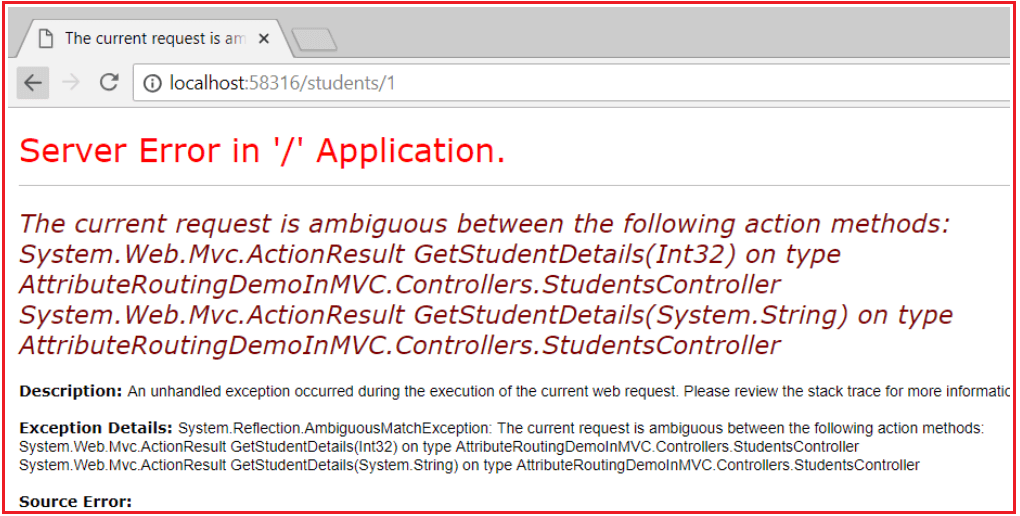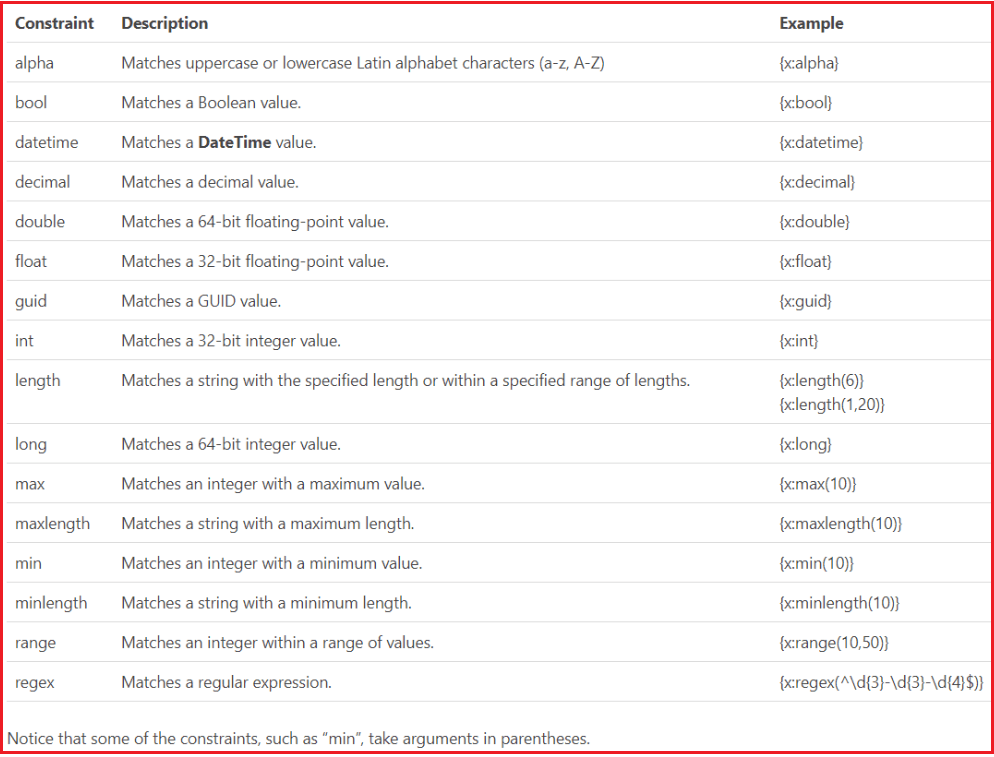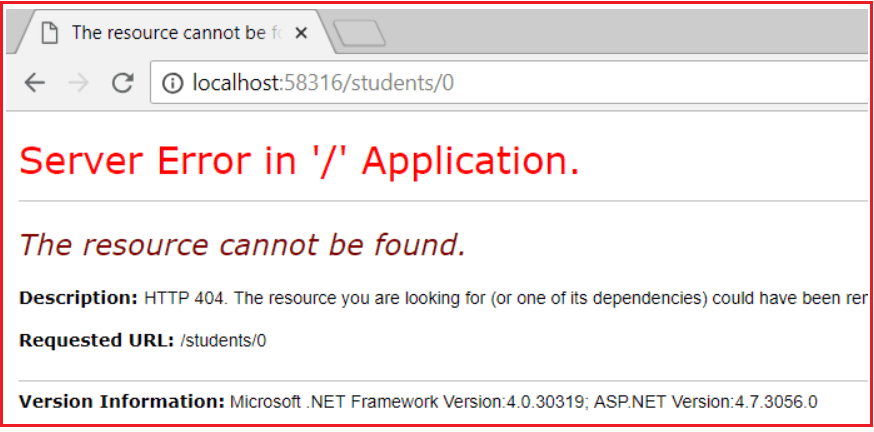# Route Constraints in ASP.NET MVC Attribute Routing

## Route Constraints in ASP.NET MVC Attribute Routing

In this article, I am going to discuss the Route Constraints in ASP.NET MVC Attribute Routing. We are going to work with the same example that we started in the Attribute Routing article of this article series. Please read Attribute Routing before proceeding to this article.

##### Route Constraints in ASP.NET MVC Attribute Routing

Route Constraints in ASP.NET MVC Attribute Routing are nothing but a set of rules that can be applied to the route parameters. By using the “:” symbol we can applied Route Constraints to the Route Parameters. Let us understand How to use the Route Constraints with one example. First, modify the Students Controller as shown below.

```namespace AttributeRoutingDemoInMVC.Controllers
{
[RoutePrefix("students")]
public class StudentsController : Controller
{
static List<Student> students = new List<Student>()
{
new Student() { Id = 1, Name = "Pranaya" },
new Student() { Id = 2, Name = "Priyanka" },
new Student() { Id = 3, Name = "Anurag" },
new Student() { Id = 4, Name = "Sambit" }
};

[HttpGet]
[Route("{studentID}")]
public ActionResult GetStudentDetails(int studentID)
{
Student studentDetails = students.FirstOrDefault(s => s.Id == studentID);
return View(studentDetails);
}
}
}
```
###### GetStudentDetails.cshtml

Now add the GetStudentDetails view and then copy and paste the below code into it.

```@model AttributeRoutingDemoInMVC.Models.Student
@{
ViewBag.Title = "GetStudentDetails";
}
<h2>GetStudentDetails</h2>
<div>
<h4>Student</h4>
<hr />
<dl class="dl-horizontal">
<dt>
@Html.DisplayNameFor(model => model.Name)
</dt>

<dd>
@Html.DisplayFor(model => model.Name)
</dd>
<dt>
@Html.DisplayNameFor(model => model.Id)
</dt>

<dd>
@Html.DisplayFor(model => model.Id)
</dd>
</dl>
</div>
```

Now If you navigate to /students/1 URL, then GetStudentDetails(int studentID) action method is executed and we get the details of the student whose id is 1 as expected. Let’s change our requirement, in addition to retrieving the student details by “student Id”, we also want to retrieve the student details by “student Name“. So let’s add another GetStudentDetails() method by taking a string parameter as shown below.

```namespace AttributeRoutingDemoInMVC.Controllers
{
[RoutePrefix("students")]
public class StudentsController : Controller
{
static List<Student> students = new List<Student>()
{
new Student() { Id = 1, Name = "Pranaya" },
new Student() { Id = 2, Name = "Priyanka" },
new Student() { Id = 3, Name = "Anurag" },
new Student() { Id = 4, Name = "Sambit" }
};

[HttpGet]
[Route("{studentID}")]
public ActionResult GetStudentDetails(int studentID)
{
Student studentDetails = students.FirstOrDefault(s => s.Id == studentID);
return View(studentDetails);
}

[HttpGet]
[Route("{studentName}")]
public ActionResult GetStudentDetails(string studentName)
{
Student studentDetails = students.FirstOrDefault(s => s.Name == studentName);
return View(studentDetails);
}
}
}
```

At this point build the solution, and navigate to the following URI’s
http://localhost:58316/students/1
http://localhost:58316/students/Pranaya

In both the case, you will get the following error.This is because the ASP.NET MVC Framework does not know or does not identify which version of the GetStudentDetails() action method to use when the request comes. This is where constraints play a very important role. If an integer is specified in the URL (/students/1), then we want to execute the GetStudentDetails(int studentId) action method which has an integer parameter. If a string is specified in the URI (/students/Pranaya), then we want to execute the GetStudentDetails(string studentName) action method which has the string parameter

##### Applying Attribute Route Constraints in ASP.NET MVC

This can be very easily achieved using Attribute Route Constraints in the ASP.NET MVC application. To specify attribute route constraint, the syntax is “{parameter:constraint}“. With these constraints in place, if the parameter segment in the URL is an integer, then GetStudentDetails(int studentId) action method with integer parameter is invoked, and if it is a string then GetStudentDetails(string studentName) action method with string parameter is invoked.

Let’s modify the Student Controller to use Attribute Route Constraints as shown below to achieve the above requirements.

```namespace AttributeRoutingDemoInMVC.Controllers
{
[RoutePrefix("students")]
public class StudentsController : Controller
{
static List<Student> students = new List<Student>()
{
new Student() { Id = 1, Name = "Pranaya" },
new Student() { Id = 2, Name = "Priyanka" },
new Student() { Id = 3, Name = "Anurag" },
new Student() { Id = 4, Name = "Sambit" }
};

[HttpGet]
[Route("{studentID:int}")]
public ActionResult GetStudentDetails(int studentID)
{
Student studentDetails = students.FirstOrDefault(s => s.Id == studentID);
return View(studentDetails);
}

[HttpGet]
[Route("{studentName:alpha}")]
public ActionResult GetStudentDetails(string studentName)
{
Student studentDetails = students.FirstOrDefault(s => s.Name == studentName);
return View(studentDetails);
}
}
}
```

Now build the solution, and navigate to the following two URIs and see everything is working as expected.
http://localhost:58316/students/1
http://localhost:58316/students/Pranaya

Please note that “alpha” stands for uppercase or lowercase alphabet characters. Along with int and alpha, we also have constraints like decimal, double, float, long, bool, etc. as shown in the below image.##### Example:

If you want GetStudentDetails(int studentId) action method to be mapped to URI /students/{studentId}, only if studentId is a number greater than ZERO, then use the “min” constraint as shown below.

```[Route("{studentID:int:min(1)}")]
public ActionResult GetStudentDetails(int studentID)
{
Student studentDetails = students.FirstOrDefault(s => s.Id == studentID);
return View(studentDetails);
}
```

With the above change, if you specify a positive number like 1 in the URI, then it will be mapped to GetStudentDetails(int studentID) action method as expected

###### /students/1

However, if you specify 0 or a negative number, you will get an error. For example, if you specify 0 as the value for studentID in the URI,

http://localhost:58316/students/0

You will get the below errorAlong with the “min” constraint, you can also specify the “max” constraint as shown below. For example, if you want the studentID value in the URI to be between 1 and 3 inclusive, then you can specify both “min” and “max” constraints as shown below.

```[HttpGet]
[Route("{studentID:int:min(1):max(3)}")]
public ActionResult GetStudentDetails(int studentID)
{
Student studentDetails = students.FirstOrDefault(s => s.Id == studentID);
return View(studentDetails);
}
```

The above example can also be achieved using just the “range” attribute as shown below

```[HttpGet]
[Route("{studentID:int:range(1,3)}")]
public ActionResult GetStudentDetails(int studentID)
{
Student studentDetails = students.FirstOrDefault(s => s.Id == studentID);
return View(studentDetails);
}
```

1.2.